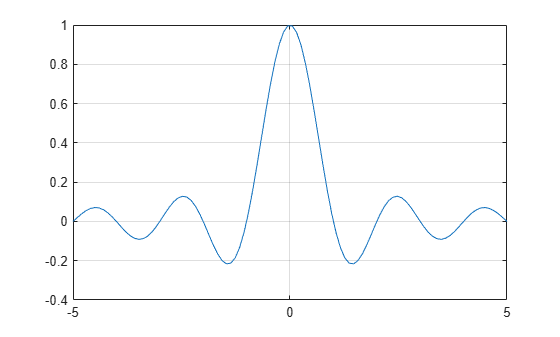# The Sinc Function

The `sinc` function computes the mathematical sinc function for an input vector or matrix $x$. Viewed as a function of time, or space, the sinc function is the inverse Fourier transform of the rectangular pulse in frequency centered at zero, with width $2\pi$ and unit height:

`$\mathrm{sinc}\text{\hspace{0.17em}}\mathit{x}=\frac{1}{2\pi }{\int }_{-\pi }^{\pi }{\mathit{e}}^{\mathit{j}\omega \mathit{x}}\text{\hspace{0.17em}}\mathit{d}\omega =\left\{\begin{array}{cc}\frac{\mathrm{sin}\text{\hspace{0.17em}}\pi \mathit{x}}{\pi \mathit{x}},& \mathit{x}\ne 0,\\ 1,& \mathit{x}=0.\end{array}$`

To plot the sinc function for a linearly spaced vector with values ranging from $-5$ to $5$, use these commands:

```x = linspace(-5,5); y = sinc(x); plot(x,y) grid```